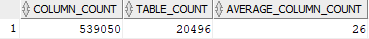## Find the number of columns in tables in Oracle database

Article for:

Query below lists:

(A) basic statistics of number of columns in all tables accessible to the current user in Oracle database

(B) basic statistics of number of columns in all tables in Oracle database

Query was executed under the Oracle9i Database version.

## Query

A. Tables accessible to the current user

``````select count(*) as column_count,
count(distinct t.table_name) as table_count,
round(count(*) / count(distinct t.table_name))
as average_column_count
from sys.all_tables t
inner join sys.all_tab_columns col on t.owner = col.owner
and t.table_name = col.table_name;
``````

B. If you have privilege on dba_tables and dba_tab_columns

``````select count(*) as column_count,
count(distinct t.table_name) as table_count,
round(count(*) / count(distinct t.table_name))
as average_column_count
from sys.dba_tables t
inner join sys.dba_tab_columns col on t.owner = col.owner
and t.table_name = col.table_name;
``````

## Columns

• column_count - number of columns in tables in a database
• table_count - number of tables in a database
• average_column_count - average number of columns in a table in a database

## Rows

Query returns one row

## Sample results0